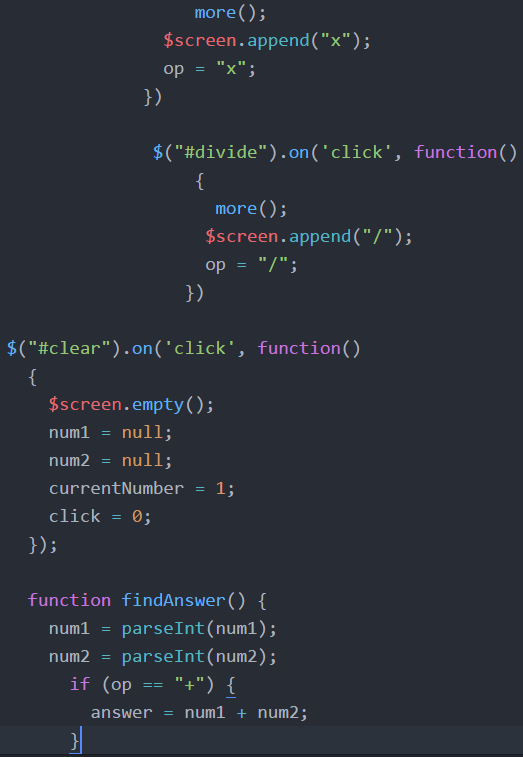# Calculator Project – Return

Our calculator is calculating! But what if we want to do even more. What if adding two numbers together just doesn’t cut it. We can’t just create an infinite number of variables named num3, num4, num1000000000, etc., just in case the user wants to use that many different numbers in their equation.

To accommodate more operating going on we just need to make a minor adjustment. If an operator is pressed after there are two numbers, it will go ahead and find the answer. Then that answer becomes the first number.

If we just kept writing numbers and operators to the page, it would get overrun. The numbers would eventually go off the screen. To prevent that, we just empty the screen if they are going to continue. Then the answer is written on the screen as the first number, num1, and the operator goes up with it. The next number entered is num2 and everything carries on as normal.

When clear is hit, num1 goes back to null just as before. But when equals is hit, the answer becomes num1 so that the work can continue.

Since I was thinking about numbers running off the screen. I added a little safeguard to try to prevent the numbers from getting so big that they would go off the screen. I just picked 8 digits as my number. I created a variable called click. When a number is clicked, it adds one. Once click is greater than 8, it doesn’t allow it to type in another number.

To stop the code from running, I use “return.” Return says stop running this function. It jumps you out of the function.

function returnPractice() { if (click > 8) {return;}

alert (‘This will never show if click is greater than 8.’); }

You could simply add code to “return” if the numbers filled the screen, maybe if click equaled 15, but I wanted to leave room for the calculation. It’s maybe not a perfect solution, but it’s good enough for me right now. When they hit an operator, I lower click by 5 to give them some more room for more numbers. Then if click is high enough, I empty the screen before writing the answer on the screen.

BTW, in the code above, alert pops a box onto the screen. Give it a try.

You can see this lesson online on Youtube.

Here is most of the code. I do declare click as a variable at the top of the code and set it equal to zero to begin.

var click = 0;When someone clicks on a number, it adds one to the variable click by using the shorthand ++. That means click = click + 1. If click is more than 8, then it gets out of there and nothing is written on the screen. If we are working on our first number and nothing is saved as num1 yet, we save that number as number 1; otherwise, we tag the new number onto num1. If 1 and then 2 was pressed, the new number is 12.The function called more is run every time an operator is pressed. Instead of copying the code into each operation, we pull it out so that any time a change is made, it is applied everywhere. It checks how big click is getting. If we already have two numbers, then it finds the answer and writes the answer to the screen. If we’re on the first number, then when an operator is pushed, we start working on the second number.When clear is clicked, we make sure that click goes back to zero and we start working on the first number. You may remember that the parseInt code is about turning num1 into a real number. When we write num1 + num2, then 1 and 2 become 12, as if they were S and O writing SO. They are taken in as a type of variable called a string. This turns them into integers, into numbers, so that 1 + 2 = 3 instead of 12.We set num1 to the answer when we want to keep adding or multiplying or whatever. The answer because the first number and then we choose an operator and then enter in one more number before the next calculation. In this final bit of code, we click on equal. If click is larger than 8, we empty off the screen before writing the answer. Those two little lines of code about answer length, we’ll get to next lesson.

You can watch the lesson.